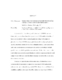## ESTIMATING UNKNOWN KNOTS IN PIECEWISE LINEAR-LINEAR LATENT GROWTH MIXTURE MODELS

 dc.contributor.advisor Hancock, Gregory R en_US dc.contributor.advisor Harring, Jeffrey R en_US dc.contributor.author Kohli, Nidhi en_US dc.contributor.department Measurement, Statistics and Evaluation en_US dc.contributor.publisher Digital Repository at the University of Maryland en_US dc.contributor.publisher University of Maryland (College Park, Md.) en_US dc.date.accessioned 2011-10-08T06:04:35Z dc.date.available 2011-10-08T06:04:35Z dc.date.issued 2011 en_US dc.description.abstract A piecewise linear-linear latent growth mixture model (LGMM) combines features of a piecewise linear-linear latent growth curve (LGC) model with the ideas of latent class methods all within a structural equation modeling (SEM) context. A piecewise linear-linear LGMM is an appropriate framework for analyzing longitudinal data that come from a mixture of two or more subpopulations (i.e., latent classes) where each latent class incorporates a separate growth trajectory corresponding to multiple growth phases from which repeated measurements arise. The benefit of the model is that it allows the specification of each growth phase to conform to a particular form of overall change process within each latent class thereby making these models flexible and useful for substantive researchers. There are two main objectives of this current study. The first objective is to demonstrate how the parameters of a piecewise linear-linear LGMM, including the unknown knot, can be estimated using standard SEM software. A series of Monte Carlo simulations empirically investigated the ability of piecewise linear-linear LGMMs to recover true (known) growth parameters of distinct populations. Specifically, the current research compared the performance of the piecewise linear-linear LGMM under different manipulated conditions of 1) sample size, 2) class mixing proportions, 3) class separation of location of knot, 4) the mean of the slope growth factor of the second phase, 5) the variance of the slope growth factor of the second phase, and 6) residual variance of the observed variables. The second objective is to address the issue of model mis-specification. It is important to analyze this issue because applied researchers have to make model selection decisions. Therefore, the current research examined the possibility of extracting spurious latent classes. To achieve this objective 1-, 2-, and 3-class piecewise linear-linear LGMMs were fit to data sets generated under different manipulated conditions using a 2-class piecewise linear-linear LGMM as a population model. The number of times the correct model (i.e., 2-class piecewise linear-linear LGMM) was preferred over incorrect models (i.e., 1- and 3-class piecewise linear-linear LGMMs) using the Bayesian Information Criterion (BIC) was examined. Results suggested that the recovery of model parameters, specifically, the variances of growth factors were generally poor. In addition, none of the manipulated conditions were systematically related to the outcome measures, parameter bias and variability index of parameter bias. Furthermore, among all the manipulated conditions, the residual variance of observed variable had the strongest statistically significant effect on both the model convergence rate and the model selection rate. Other manipulated conditions that had an impact on the model convergence rate and/or the model selection rate were the growth factor mean of slope of the second phase, the growth factor variance of slope of the second phase, and the class mixing proportion. The manipulated conditions whose levels had no influence on either the model convergence rate or the model selection rate were sample size and the class separation of location of knot. en_US dc.identifier.uri http://hdl.handle.net/1903/11973 dc.subject.pqcontrolled Educational tests & measurements en_US dc.subject.pqcontrolled Quantitative psychology and psychometrics en_US dc.subject.pquncontrolled Finite Mixtures en_US dc.subject.pquncontrolled Knot en_US dc.subject.pquncontrolled Latent growth curve models en_US dc.subject.pquncontrolled Piecewise en_US dc.title ESTIMATING UNKNOWN KNOTS IN PIECEWISE LINEAR-LINEAR LATENT GROWTH MIXTURE MODELS en_US dc.type Dissertation en_US
##### Original bundle
Now showing 1 - 1 of 1